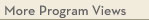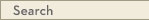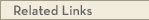# University Catalogs

Duluth campusDuluth Campus

# Statistics Minor

Mathematics & Statistics
Swenson College of Science and Engineering
• Program Type: Undergraduate minor related to major
• Requirements for this program are current for Fall 2021
• Required credits in this minor: 21 to 27
The minor in statistics is based on the completion of traditional mathematics courses including Calculus and Linear Algebra, a required introductory statistics course, one advanced course in a traditional statistics area, and two additional, advanced elective statistics courses. The minor in statistics certifies a student's quantitative, problem-solving, and critical thinking skills.
Program Delivery
This program is available:
• via classroom (the majority of instruction is face-to-face)
Minor Requirements
1. The Statistics minor is not allowed with a Mathematics major or with a Statistics major. 2. Students may not receive both a minor in Mathematics and a minor in Statistics.
Mathematics Requirement (8 - 9 cr)
Calculus I
MATH 1290 - Calculus for the Natural Sciences [LE CAT2, LOGIC & QR] (5.0 cr)
or MATH 1296 - Calculus I [LE CAT, LOGIC & QR] (5.0 cr)
Students choosing MATH 3280 will be required to complete the prerequisite course MATH 1297 Calculus II.
MATH 3280 - Differential Equations with Linear Algebra (4.0 cr)
or MATH 3326 - Vectors and Matrices (3.0 cr)
Calculus II (optional) (5 cr)
Students choosing MATH 3280, STAT 3411, 3612, or 5511 will be required to complete the prerequisite course MATH 1297 Calculus II.
MATH 1297 - Calculus II [LOGIC & QR] (5.0 cr)
Statistics Requirements (6 - 7 cr)
Intro Statistics
Students choosing STAT 3411 will be required to complete the prerequisite course MATH 1297 Calculus II.
STAT 3411 - Engineering Statistics (3.0 cr)
or STAT 3611 - Introduction to Probability and Statistics (4.0 cr)
Students choosing STAT 3612 or 5511 will be required to complete the prerequisite course MATH 1297 Calculus II.
STAT 3612 - Introduction to Probability and Statistics II (3.0 cr)
or STAT 5411 - Analysis of Variance (3.0 cr)
or STAT 5511 - Regression Analysis (3.0 cr)
Statistics Electives (6 cr)
Each elective course must be 3 credits or more. Neither STAT 3411 nor 3611 can be counted as Statistics Electives. Any of STAT 3612, 5411, and 5511, which are not counted in the Statistics Requirement category, may count towards the Statistics Electives.
Take 2 or more course(s) totaling 6 or more credit(s) from the following:
· STAT 3xxx
· STAT 4xxx
· STAT 5xxxView college catalog(s): · Swenson College of Science and Engineering View checkpoint chart: · Statistics Minor View PDF Version:Search Programs Search University CatalogsOne Stop for tuition, course registration, financial aid, academic calendars, and moreMATH 1290 - Calculus for the Natural Sciences (LE CAT2, LOGIC & QR)
 Credits: 5.0 [max 5.0] Course Equivalencies: Math1290/1296/1596 Grading Basis: A-F or Aud Typically offered: Every Fall & Spring
Differential and integral calculus needed for modeling in earth and life sciences. Computational software. Not intended for students in mathematics, engineering, or physical sciences. prereq: Math ACT 27 or higher or a grade of at least C- in Math 1250 or department consent
MATH 1296 - Calculus I (LE CAT, LOGIC & QR)
 Credits: 5.0 [max 5.0] Course Equivalencies: Math1290/1296/1596 Grading Basis: A-F or Aud Typically offered: Every Fall, Spring & Summer
First part of a standard introduction to calculus of functions of a single variable. Limits, continuity, derivatives, integrals, and their applications. prereq: Math ACT 27 or higher or a grade of at least C- in Math 1250 or department consent
MATH 3280 - Differential Equations with Linear Algebra
 Credits: 4.0 [max 4.0] Grading Basis: A-F or Aud Typically offered: Every Fall, Spring & Summer
First, second, and higher order equations; series methods; Laplace transforms; systems; software; modeling applications; introduction to vectors; matrix algebra, eigenvalues. prereq: A grade of at least C- in 1297 or 1597
MATH 3326 - Vectors and Matrices
 Credits: 3.0 [max 3.0] Grading Basis: A-F or Aud Typically offered: Every Fall
Solving systems of linear equations; matrix algebra; determinants; an introduction to vector spaces, subspaces, linear independence, span, basis; coordinates, matrix transformations, eigenvalues, eigenvectors, matrix factorizations and applications to computer graphics. prereq: 1296 with a grade of C- or better
MATH 1297 - Calculus II (LOGIC & QR)
 Credits: 5.0 [max 5.0] Course Equivalencies: Math 1597/1297 Grading Basis: A-F or Aud Typically offered: Every Fall, Spring & Summer
Second part of a standard introduction to calculus. Vectors, applications of integrals, transcendental functions, series, and multivariable functions and partial derivatives. prereq: A grade of at least C- in 1290 or 1296 or 1596
STAT 3411 - Engineering Statistics
 Credits: 3.0 [max 3.0] Grading Basis: A-F or Aud Typically offered: Every Fall & Spring
Statistical considerations in data collection and experimentation. Descriptive statistics, least squares, elementary probability distributions, confidence intervals, significance tests, and analysis of variance as applied analysis of engineering data. prereq: MATH 1297 with a grade of C- or better, cannot be applied to a math or statistics major
STAT 3611 - Introduction to Probability and Statistics
 Credits: 4.0 [max 4.0] Grading Basis: A-F or Aud Typically offered: Every Fall, Spring & Summer
Basic probability, including combinatorial methods, random variables, mathematical expectation. Binomial, normal, and other standard distributions. Moment-generating functions. Basic statistics, including descriptive statistics and sampling distributions. Estimation and statistical hypothesis testing. prereq: A grade of at least C- in Math 1290 or Math 1296
STAT 3612 - Introduction to Probability and Statistics II
 Credits: 3.0 [max 3.0] Grading Basis: A-F or Aud Typically offered: Every Fall & Spring
An introduction to statistics. Sample distributions, point and interval estimation, hypothesis testing, linear regression, one- and two-way analysis of variance, goodness-of-fit and non-parametric statistics. prereq: 3611 and Math 1297 or equivalent or instructor consent
STAT 5411 - Analysis of Variance
 Credits: 3.0 [max 3.0] Typically offered: Every Fall
Analysis of variance techniques as applied to scientific experiments and studies. Randomized block designs, factorial designs, nesting. Checking model assumptions. Using statistical computer software. prereq: 3411 or 3611; a grade of C- or better is required in all prerequisite courses
STAT 5511 - Regression Analysis
 Credits: 3.0 [max 3.0] Prerequisites: 3611, Math 3280 or Math 4326, a grade of C- or better in is required in all prerequisite courses Grading Basis: A-F or Aud Typically offered: Every Fall & Spring
Simple, polynomial, and multiple regression. Matrix formulation of estimation, testing, and prediction in linear regression model. Analysis of residuals, model selection, transformations, and use of computer software. prereq: 3611, Math 3280 or Math 4326, a grade of C- or better in is required in all prerequisite courses